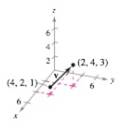Chapter 11.2, Problem 45E

Chapter
Section
Textbook Problem

# Finding the Component Form of a Vector in Space In Exercises 47 and 48. (a) find the component form of the vector v,(b) write the vector living standard unit vector notation, and(c) sketch the vector with its initial point at the origin.(a)

To determine
The component form of the vector v provided in the graph.

Explanation

Given: The graph

Explanation: Consider the provided the graph. From the graph, the initial point is vi=(4,2,1), and the terminal point is vt=(2,4,3).

Now the required vector v is given by,

v=terminal point-initial point,

Thus,

v=vtvi

(b)

To determine
The Standard unit notation of the vector.

(c)

To determine

To graph: The vector whose initial point lies at the origin.

### Still sussing out bartleby?

Check out a sample textbook solution.

See a sample solution

#### The Solution to Your Study Problems

Bartleby provides explanations to thousands of textbook problems written by our experts, many with advanced degrees!

Get Started

#### Simplify the expressions in Exercises 97106. 32/331/6

Finite Mathematics and Applied Calculus (MindTap Course List)

#### Perform each operation and simplify: a. (x2+1)(12x1/2)x1/2(2x)(x2+1)2 b. 3xx+2+3x+2

Applied Calculus for the Managerial, Life, and Social Sciences: A Brief Approach

#### In problems 23-58, perform the indicated operations and simplify. 24.

Mathematical Applications for the Management, Life, and Social Sciences

#### n=1n2n3+1

Calculus: Early Transcendental Functions (MindTap Course List)

#### Find for x = 3t2 + 1, y = t6 + 6t5. t4 + 5t3 4t3 + 15t2

Study Guide for Stewart's Single Variable Calculus: Early Transcendentals, 8th